## Arithmetic With Complex Numbers

### Learning Outcomes

• Identify the difference between an imaginary number and a complex number
• Identify the real and imaginary parts of a complex number
• Plot a complex number on the complex plane
• Perform arithmetic operations on complex numbers
• Graph physical representations of arithmetic operations on complex numbers as scaling or rotation
• Generate several terms of a recursive relation
• Determine whether a complex number is part of the set of numbers that make up the Mandelbrot set

## Complex Numbers


The numbers you are most familiar with are called real numbers. These include numbers like 4, 275, -200, 10.7, ½, π, and so forth. All these real numbers can be plotted on a number line. For example, if we wanted to show the number 3, we plot a point:

To solve certain problems like $x^{2}=–4$, it became necessary to introduce imaginary numbers.

### Imaginary Number i

The imaginary number i is defined to be $i=\sqrt{-1}$.

Any real multiple of i, like 5i, is also an imaginary number.

### Example

Simplify $\sqrt{-9}$.

We can separate $\sqrt{-9}$ as $\sqrt{9}\sqrt{-1}$. We can take the square root of 9, and write the square root of $-1$ as i.

$\sqrt{-9}=\sqrt{9}\sqrt{-1}=3i$

A complex number is the sum of a real number and an imaginary number.

### Complex Number

A complex number is a number $z=a+bi$, where

• a and b are real numbers
• a is the real part of the complex number
• b is the imaginary part of the complex number

To plot a complex number like $3-4i$, we need more than just a number line since there are two components to the number. To plot this number, we need two number lines, crossed to form a complex plane.

### Complex Plane

In the complex plane, the horizontal axis is the real axis and the vertical axis is the imaginary axis.### Example

Plot the number $3-4i$ on the complex plane.

The real part of this number is 3, and the imaginary part is $-4$. To plot this, we draw a point 3 units to the right of the origin in the horizontal direction and 4 units down in the vertical direction.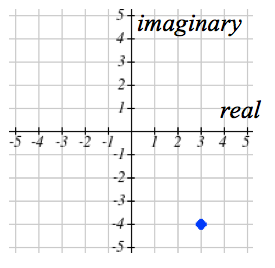### Try It

Because this is analogous to the Cartesian coordinate system for plotting points, we can think about plotting our complex number $z=a+bi$ as if we were plotting the point (a, b) in Cartesian coordinates. Sometimes people write complex numbers as $z=x+yi$ to highlight this relation.

## Arithmetic on Complex Numbers

Before we dive into the more complicated uses of complex numbers, let’s make sure we remember the basic arithmetic involved. To add or subtract complex numbers, we simply add the like terms, combining the real parts and combining the imaginary parts.

### Example

Add $3-4i$ and $2+5i$.

Adding $(3-4i)+(2+5i)$, we add the real parts and the imaginary parts.

$3+2-4i+5i$

$5+i$

### Try It

Subtract $2+5i$ from $3-4i$.

In the following video, we present more worked examples of arithmetic with complex numbers.

When we add complex numbers, we can visualize the addition as a shift, or translation, of a point in the complex plane.

### Example

Visualize the addition $3-4i$ and $-1+5i$.

The initial point is $3-4i$. When we add $-1+3i$, we add $-1$ to the real part, moving the point 1 units to the left, and we add 5 to the imaginary part, moving the point 5 units vertically. This shifts the point $3-4i$ to $2+1i$.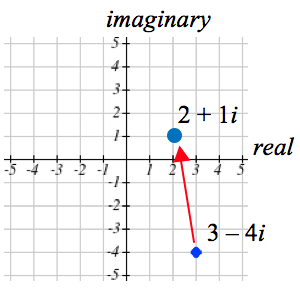### Try It

We can also multiply complex numbers by a real number, or multiply two complex numbers.

### Example

Multiply: $4\left(2+5i\right)$

To multiply the complex number by a real number, we simply distribute as we would when multiplying polynomials.

Distribute and simplify.

$4(2+5i)\\\,\,\,= 4\cdot2+4\cdot5i\\\,\,\,=8+20i$

### Example

Multiply: $(2+5i)(4+i)$.

$\begin{array}{l}\left(2+5i\right)\left(4+i\right)\,\,\,\,\,\,\,\,\,\,\,\,\,\,\,\,\,\,\,\,\,\,\,\,\,\,\,\,\,\,\,\,\,\,\,\,\,\text{Expand.}\\=8+20i+2i+5i^{2}\,\,\,\,\,\,\,\,\,\,\,\,\,\,\,\,\,\,\,\,\,\,\,\,\,\text{Since }i=\sqrt{-1},i^{2}=-1\\=8+20i+2i+5\left(-1\right)\,\,\,\,\,\,\,\,\,\,\,\,\,\,\,\text{Simplify.}\\=3+22i\end{array}$

### Try It

Multiply $3-4i$ and $2+3i$.

To understand the effect of multiplication visually, we’ll explore three examples.

### Example

Visualize the product $2(1+2i)$.

Multiplying we’d get

\begin{align}&2\cdot1+2\cdot2i\\&=2+4i\\\end{align}

Notice both the real and imaginary parts have been scaled by 2. Visually, this will stretch the point outwards, away from the origin.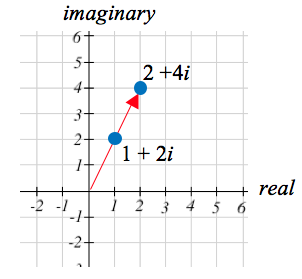### Example

Visualize the product $i\left(l+2i\right)$.

Multiplying, we’d get

\begin{align}&i\cdot1+i\cdot2i\\&=i+2{{i}^{2}}\\&=i+2(-1)\\&=-2+i\\\end{align}

In this case, the distance from the origin has not changed, but the point has been rotated about the origin, 90° counter-clockwise.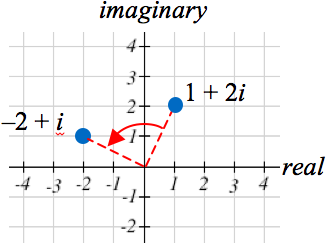### Try It

Multiply $3-4i$ and $2+3i$.

### Example

Visualize the result of multiplying $1+2i$ by $1+i$. Then show the result of multiplying by $1+i$ again.

Multiplying $1+2i$ by $1+i$,

$-4+2i$

\begin{align}&(1+2i)(1+i)\\&=1+i+2i+2{{i}^{2}}\\&=1+3i+2(-1)\\&=-1+3i\\\end{align}

Multiplying by $1+i$ again,

\begin{align}&(-1+3i)(1+i)\\&=-1-i+3i+3{{i}^{2}}\\&=-1+2i+3(-1)\\&=-4+2i\\\end{align}

If we multiplied by $1+i$ again, we’d get $–6–2i$. Plotting these numbers in the complex plane, you may notice that each point gets both further from the origin, and rotates counterclockwise, in this case by 45°.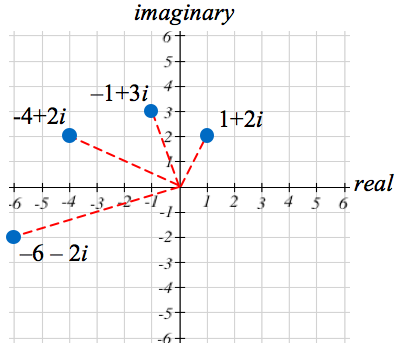In general, multiplication by a complex number can be thought of as a scaling, changing the distance from the origin, combined with a rotation about the origin.

### Try It

The following video presents more examples of how to visualize the results of arithmetic on complex numbers.

In the following video, we present more worked examples of arithmetic with complex numbers.

1. Portions of this section are remixed from Precalculus: An Investigation of Functions by David Lippman and Melonie Rasmussen. CC-BY-SA